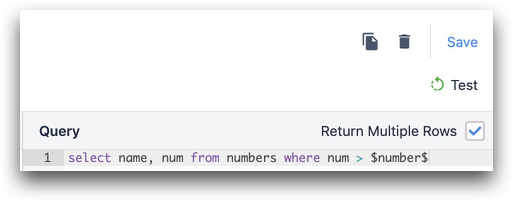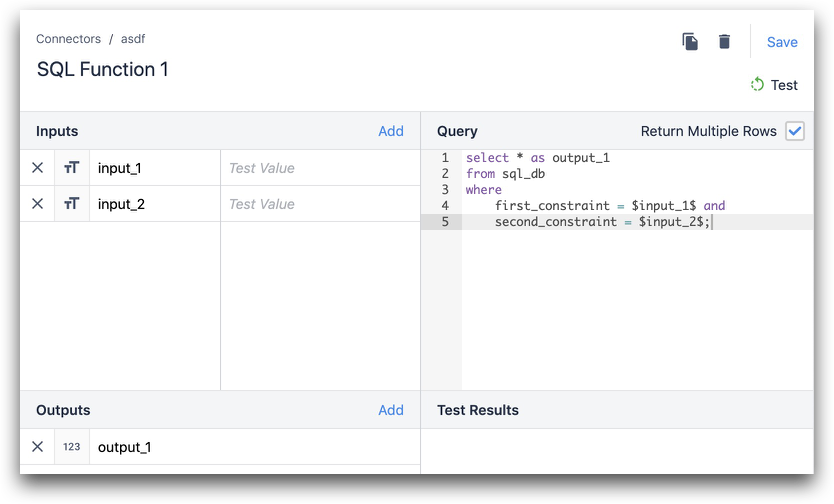• English
Common SQL Connector Functions

# Common SQL Connector Functions

Article Summary

## Common SQL Connector Functions

This articles describes how to write some commonly used SQL functions in Tulip Connectors.

Before reading this guide, see our other tutorial for creating your first SQL connector function in Tulip

Listed below are a few simple and commonly used SQL Connector Functions that you can utilize in your SQL queries:

## SELECT Statement:

Consider a scenario where you would like to view details about a particular Work Order that is stored in your MES/ERP database.  The SELECT statement can help us with this task:

``````SELECT * FROM table_in_your_database
``````

This will return all rows and columns from your table.

You can return either a single row or multiple rows. If you want to return a single row, add conditions or limits to your query. Tulip inputs are commonly used in this case. In the example below work_order_number is a Tulip function input.

``````SELECT * FROM table_in_your_database
WHERE column_1 = \$work_order_number\$
``````

If you want to return multiple rows, make sure to check the box under "Return Multiple Rows?"Returning Data

If the database column names match the output names that you have defined in the connector function, Tulip will automatically associate the query results with the function outputs. Example: Tulip output is output_1 and database column is also output_1

If the column names in your database differ from what you would like to use in Tulip you must use an alias to make the proper association between the two.

In the example below column_1 is from the database and output_1 is the Tulip output.

``````SELECT column_1 as output_1 FROM table_in_your_database
where
first_constraint = \$input_1\$ and
second_constraint = \$input_2\$;
``````## INSERT Statement:

Consider a scenario where you would like to insert into your MES/ERP with data from a Tulip App.  You would utilize a simple INSERT function to achieve this task.  Here is a sample of what this function looks like in SQL:

``````INSERT INTO table_in_your_database
``````

Now let's break down each part of this function:

Identify the table in your database

``````INSERT INTO table_in_your_database
``````

Choose the columns in your database

``````(username, user_id, product_id)
``````

Define the values from Tulip

``````VALUES (\$username\$, \$user_id\$, \$product_id\$)
``````

## UPDATE Statement:

Consider a scenario where you would like to update your MES/ERP with data from a Tulip App, using a Work Order as a key.  You would utilize the UPDATE function, as shown below:

``````UPDATE table_in_your_database
SET column_1 = \$input_1\$,
column_2 = \$input_2\$
WHERE work_order = \$work_order\$
``````

Now let's break down each part of this function:

Identify the table in your database

``````UPDATE table_in_your_database
``````

Define the columns to update with Tulip data

``````SET column_1 = \$input_1\$,
column_2 = \$input_2\$
``````

Use the Work Order as a condition

``````WHERE work_order = \$work_order\$
``````